# Assignments

1. GPA score
The data for this assignment are listed in the Excel file GPA score.
The admissions officer of a university is trying to develop a formal system of deciding which students to admit to the university. She believes that determinants of success include the standard variables: high school grades and SAT scores. However, she also believes that students who have participated in extracurricular activities are more likely to succeed than those who have not. To investigate the issue, she randomly sampled 100 fourth-year students and recorded the following variables (see the Excel file):
Univ GPA
GPA for the first 3 years at the university (range 0-12)
HS GPA
GPA from high school (range 0-12)
SAT
SAT score (range: 400-1600)
Activities
Numbers of hours on average spent per week in organized extracurricular activities in the last year of high school.
a. Determine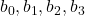in the following regression equation:
Univ GPA =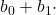HS GPA+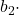SAT+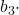Activities
and generate the usual statistics.
b. What is the coefficient of determination and interpret its value.
c. Test the overall validity of the model.
d. Interpret each of the coefficients of the independent variables.
e. Predict with 95% confidence the GPA for the first 3 years of university for a student whose high school GPA is 10, whose SAT score is 1200 and who worked an average of 2 hours per week on organized extracurricular activities in the last year of high school.
f. Estimate with 90% confidence the mean GPA for the first 3 years of university for all students whose high school GPA is 8, whose SAT score is 1100, and who worked an average of 10 hours per week organized extracurricular activities in the last year of high school.

Solution
a.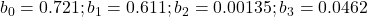. See table below.
b. The coefficient of determinations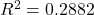; so 28.82% of the variation in university GPAs is explained by the model. The model does not fit the data very well.
c.. At least oneis not equal to zero.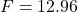,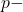value. There is enough evidence to reject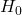and thus conclude that the model is valid.
d.; for each additional point of HS GPA Univ GPA increases on average by 0.611 provided that the other variables remain constant. Since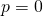, HS GPA is very relevant to University GPA.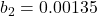; for each additional point of SAT Univ GPA increases on average by 0.00135 provided that the other variables remain constant. Since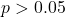, we may conclude that the SAT score may not be relevant for University GPA.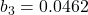; for each additional hour of Activities Univ GPA increases on average by 0.0462 provided that the other variables remain constant. Since, we may conclude that the number of hours of Activities may not be relevant for University GPA.
e. See table below. We predict that the student’s Univ GPA will fall between 4.45 and 12.00 (12 is the maximum).
f. See table below. The mean Univ GPA is estimated to lie between 6.90 and 8.22.

2.Delivery trucks
The data for this assignment are listed in the Excel file Delivery trucks.
Delivery trucks operating from a central warehouse regularly resupply stores. In an analysis of a chain’s operations, the general manager wanted to determine the factors that are related to how long it takes to unload delivery trucks.
A random sample of 50 deliveries to one store was observed. The times (in minutes) to unload the truck, the total number of boxes, the total weight (in hundreds of pounds) of the boxes and a code indicating the time of day (morning, early afternoon, late afternoon) were recorded.
a. First determine the multiple regression equation with the total number of boxes and the total weight as independent variables. Give the coefficients of both variables.
b. How well does the model fit the data? Is the model valid?

The general manager realized that another variable, the time of day, may affect unloading time. He recorded the following codes: 1 = morning, 2 = early afternoon, and 3 = late afternoon. These data can be found in the column Codes. Since these nominal variables are hard to interpret in a regression model, he introduced indicator or dummy variables.

c. Give the definition of the indicator variables based on Codes.
d. Determine the multiple regression equation with the total number of boxes, the total weight and the indicator variables as independent variables.
e. Compare the results of this model with those of the model in a. with respect to the fit of data and the validity. Which model would you prefer?
You need to use Excel.

Solution.
a. The multiple regression equation is: Time =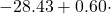Boxes +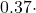Weight.
.b.The coefficient of determination equals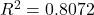. So, we may conclude that the model fits the data very well. Furthermore,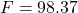with a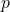-value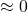, so the model is a linear model and thus valid.
c. Morning = 1 if Code = 1, Morning = 0 if not. Early afternoon = 1 if Code = 2, Early afternoon = 0 if not. Late afternoon does not need to be defined, since Morning = 0 and Early afternoon = 0 implies Late afternoon.
d. Now, the multiple equation becomes: Time =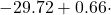Boxes +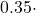Weight -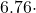Morning +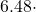Early afternoon.
e. Now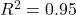. So, we may conclude that the model fits the data even better. Furthermore,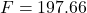with a-value, so the model is a linear model and thus valid. This enhanced model performs much better than the model in a. and is to be preferred.

3. Longevity
The data for this assignment are listed in the Excel file Longevity.
Life insurance companies are keenly interested in predicting longevity of their customers (i.e., how long will their customers live) because their premiums and profitability depend on such numbers. An actuary for one insurance company gathered data from 100 recently deceased male customers. He recorded the age at death of the customer plus the ages at death of his mother and father, the mean ages at death of his grandmothers, and the mean ages at death of his grandfathers.
a. Perform a multiple regression analysis on these data and give the regression equation.
b. The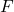-test concludes about the validity of the model. Formulate the null () and alternative (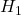) hypotheses for this-test. Give the results and explain why the model is or is not valid.
c. Interpret the coefficients of the regression equation.
d. Test the coefficients of the regression equation and explain the results.
e. Is the normality requirement satisfied?
f. Is the variance of the error variable constant?
g. Would you expect multicollinearity to be a problem?

Solution.
a. See the table below.
Longevity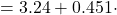Mother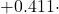Father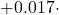Gmothers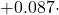Gfathers
b. The null and alternative hypotheses are: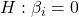; at least oneis not equal to zero. We find: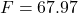and-value. There is overwhelming evidence to conclude that the model is valid.
c.; intercept, not really useful since there are no data available around zero ages.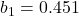; for each one year increase in the mother’s age the customer’s age increases on average by 0.451 year provided the other variables are constant (which may not be possible because of multicollinearity).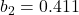; for each one year increase in the father’s age the customer’s age increases on average by 0.411 year provided the other variables are constant.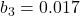; for each one year increase in the grandmother’s mean age the customer’s age increases on average by 0.017 year provided the other variables are constant.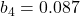; for each one year increase in the grandfather’s mean age the customer’s age increases on average by 0.087 year provided the other variables are constant.
d. The null and alternative hypotheses for each of these coefficients are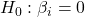and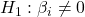Mothers: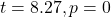Fathers: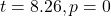Grandmothers: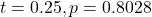Grandfathers:The ages of mothers and fathers are linearly related to the ages of their children. The other two variables are not.
e. The histogram for Frequency vs. Standard Residuals shows a normal distribution with mean approximately 0, so the normality requirement is satisfied.
f. The plot of Residuals vs. Predicted values shows that the variance of the error variable is constant.
g. The correlation matrix (Data Analysis) between Father, Mother, Gmothers and Gfathers coefficients shows correlations, some of which may cause problems with the -tests.

4. Finger length
The data for this assignment are listed in the Excel file Finger length.
A computer dating service typically asks for various pieces of information such as height, weight, income and so on. One such service requested the length of index fingers. The only plausible reason for this request is to act as an indication of the height. Women have often complained that men lie about their height. If there is a strong relationship between heights and index fingers, the information can be used to “correct” false claims about heights.
To test the relationship between the two variables researchers gathered the heights and lengths of index fingers (in centimeters) of 121 male (Gender=1) and female (Gender=0) students.
a. Compute the formula of the regression line without gender. What can you conclude about how well the model fits the data and whether the model is valid? Explain your answer.
b. Is there sufficient evidence to infer that height and length of index fingers are linearly related.
c. Now also include gender in the model and see the difference in expected height.

Solution.
a. Use Excel and get the formula of the regression line: Height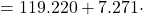Finger. The model fits the data poorly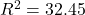. The model is valid because the-value is large with a-value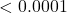which tells you that it is highly certain that there is a linear relation between Height and Finger length.
b. Excel gives as a result that the correlation between both variables is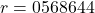, There is a moderately strong positive correlation.
c. The gender makes a great difference. Take the same finger length and see the difference in height between males and females. If the finger length is 8.2 cm then a male is assumed to be 180 cm tall and a female only 169 cm.

0